# The DFT of Finite-Length Time-Reversed Sequences

Recently I've been reading papers on underwater acoustic communications systems and this caused me to investigate the frequency-domain effects of time-reversal of time-domain sequences. I created this blog because there is so little coverage of this topic in the literature of DSP.

This blog reviews the two types of time-reversal of finite-length sequences and summarizes their discrete Fourier transform (DFT) frequency-domain characteristics.

The Two Types of Time-Reversal in DSP

In DSP the two types of finite-length sequence time-reversal are (1) what I call "Flip Time-Reversal", and (2) "Circular Time-Reversal." Figure 1 shows the two types of time-reversal of a six-sample x[n] time sequence.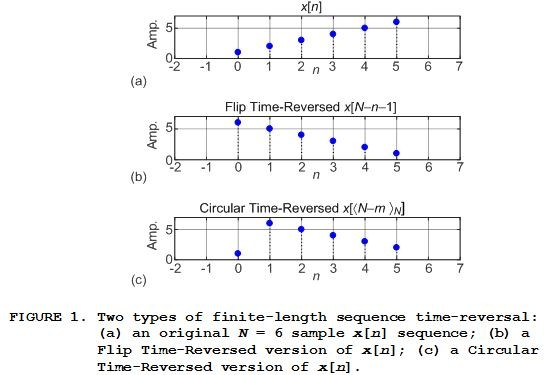Appendix A presents a review of Circular Time-Reversal and explains why the word circular is used.

The DFT of Finite-Length Time-Reversed Sequences

Here I present the principal information of this blog in the form of two tables, followed by the definitions of the algebraic notation used in those tables.

The DFTs of Flip Time-Reversed real‑ and complex‑valued time sequences are listed in Table 1.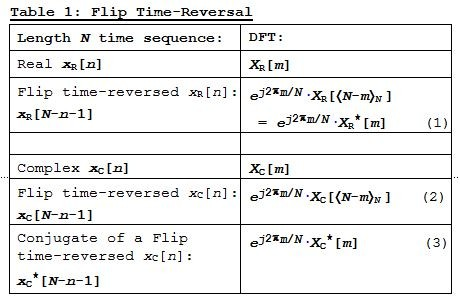The key characteristic of Flip Time-Reversal is the ej2pm/N linear phase shift term in Eqs. (1), (2), and (3). The derivations of Eqs. (1) and (2) are given in Appendix B.

The DFTs of Circular Time-Reversed real‑ and complex‑valued time sequences are listed in Table 2.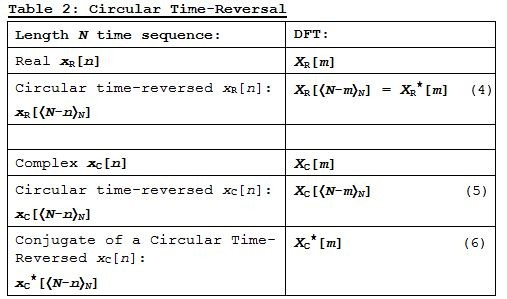The derivations of Table 1's Eq. (3) and Table 2's Eqs. (4), (5), and (6) are given in References [1‑3].

The Notation Used In Tables 1 and 2

The DFT of an arbitrary N-length x[n] time sequence is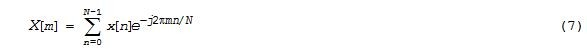where n is the time-domain integer index and m is the frequency-domain integer index. Both n and m are in the range 0, 1, 2, ..., N-1.

The algebraic notation for Flip Time Reversal of an x[n] time sequence is: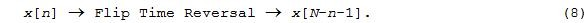An example of Eq. (8) is the sequence in Figure 1(b).

In the literature of DSP I encountered four different algebraic notations for Circular Time Reversal. The notation I choose to use here is: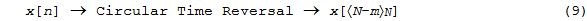where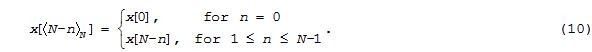An example of Eq. (10) is the sequence in Figure 1(c). The  x[<N-m>] notation in Eqs. (9) and (10) is modulo N arithmetic notation because typical algebraic derivations of time-reversed sequences require modulo N arithmetic as demonstrated in Appendix B.

Regarding the two types of finite-length sequence time-reversal, we encounter Flip Time-Reversal when we: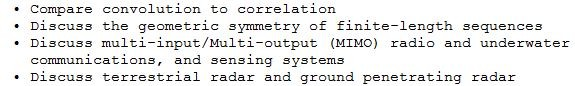We encounter Circular Time-Reversal when we: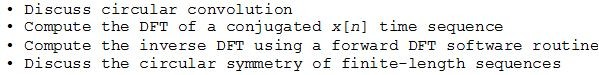Conclusion

To provide a more complete description than was currently available in one place, I've presented the two types of time-reversal of finite-length real‑ and complex‑valued sequences, and tabulated their DFT frequency-domain characteristics in Table 1 and Table 2.

Finally, I listed the instances in which we may encounter time-reversal in the field of DSP.References

 S. Mitra, "Digital Signal Processing", Fourth Edition, McGraw Hill Publishing, 2011, pp. 56, 211.

 R. Lyons, "Understanding Digital Signal Processing", Third Edition, Prentice Hall, Upper Saddle River, New Jersey, 1996, pp. 863-865.

 J. Proakis and D. Manolakis, "Digital Signal Processing-Principles, Algorithms, and Applications", Third Edition, Prentice Hall, Upper Saddle River, New Jersey, 1996, pp. 421.

Appendix A: A Brief Review of Circular Time-Reversal

In this appendix we explain why the word "circular" is used in the phrase Circular Time-Reversal. In the early days of DSP while studying the symmetric behavior of the FFT, the practitioners encountered sequences that we now call "circular time-reversed" sequences.

As an example, if

x[n] = x, x, x, x, x, x

the DFT of x[n] is a complex-valued X[m]. If we conjugate X[m] and compute its inverse DFT we obtain the following circularly-reversed y[n] time sequence:

Inverse DFT of X[m]* = y[n] = x, x, x, x, x, x.

Because all DFT operations are done over an indexing domain that is circular, we can depict the x[n] and y[n] sequences in a circular fashion as shown in Figure A-1. In Figure A-1(a), starting with x, we write the x[n] sequence counter clockwise (positive time) around a circle. In Figure A-1(b) we read the y[n] values, starting with x, going clockwise (negative time) around the circle.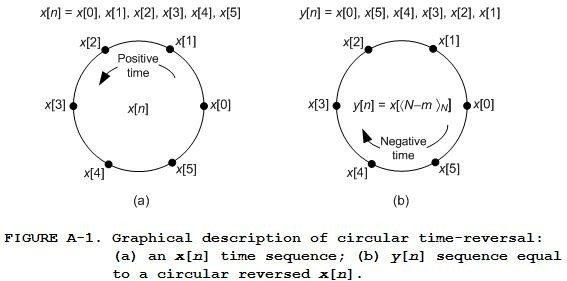Thus we refer to y[n] as a circular reversed version of sequence x[n]. The sequences in Figure A-1 correspond to the sequences in this blog's Figures 1(a) and 1(c).

Appendix B: Derivations of Eqs. (1) and (2)

Here we derive this blog's Eqs. (1) and (2). First, consider an N-sample discrete signal x[n], be it real- or complex-valued, having X[m] as its DFT as: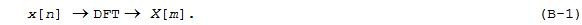Next let's think about a Flip Time-Reversed x[Nn‑1] sequence whose N-point DFT, XFTR[m], is: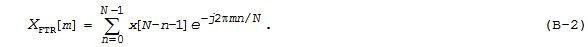We wish to find a closed-form expression for XFTR[m] that does not use a summation. If we change Eq. (B‑2)'s time indexing by letting p = Nn‑1, then in terms of p, n = Np-1. Substituting p for Nn‑1, and N-p‑1 for n, in Eq. (B‑2) we now have: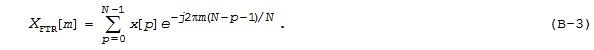Note that sequence x[p], for 0≤pN-1, in Eq. (B‑3) is equal to the original non-reversed x[n] sequence. By factoring Eq. (B‑3) we can write: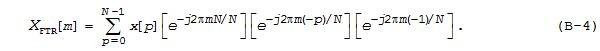In Eq. (B‑4) the first factor in brackets is equal to one for all m, and the last factor in brackets is not a function of p so we move it outside the summation to write: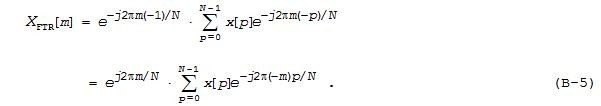Because the summation in Eq. (B‑5) is an N-point DFT with negative frequency indexing (‑m), we rewrite that equation as: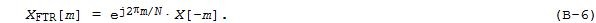Recall that all DFT operations are done over an indexing domain that is circular. When the negative-m indexing in Eq. (B‑6) is interpreted in a circular fashion using modulo N arithmetic, X[‑m] mod N in Eq. (B‑6) is equal to x[<N-m>] enabling us to write: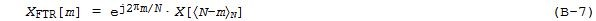verifying Table 1's Eq. (2) when x[n] is complex-valued.

Due to the symmetry of the DFT of real-valued sequences, if x[n] is real-valued then Eq. (B‑7) becomes: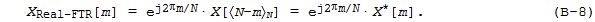verifying Table 1's Eq. (1).

[ - ]
Comment by December 25, 2019Hi Rick,

Thanks for the blog. I encountered an issue in DFT that required some sort of circular approach but it was too costly and eventually I didn't need it !!. The issue was doing DFT on a finite signal frame after using decimation filters to reduce DFT size. The initial samples equal to group delay of filters have to be discarded before DFT but that led to two issues; the group delay had to be integers at final stage and the size of frame for DFT needed to be adjusted by inserting some tail that did not exist as signal was of limited length. I noticed that removing group delay samples plus zero padding at end was enough but some people suggested circular DFT to top up sample from start towards end. That required plenty memory and logic.

Specifically as an example (LTE Prach) the frame length was 24576 samples before decimation. That required massive DFT for FPGA platform. I decimated by factor 12 to 2k size but that resulted in group delay of about 27 samples and had to discard these but from where would I get more samples at end to top up to 2k then? I tried inserting zeros or tail end of filters data and it was ok but the initial segment of filter(equal to group delay) has to be discarded as it was affecting sensitivity of signal detection.

Kaz

[ - ]
Comment by December 26, 2019Hi kaz. That sounds like an interesting signal processing problem.

Hey kaz, I seek your advice. Do you know of any online tutorials on the subject of LTE 5G that are geared for technical people, but people who are NOT long-term experts in digital communications. (The 5G descriptions I've found on the web, so far, are filled with all manner of undefined acronyms and jargon that I do not understand.) Are there any "5G For Dummies" web sites out there?  Thanks kaz!!

[ - ]
Comment by December 26, 2019Hi Rick,

We are just developing 5G network equipment and "struggling" to understand this new standard.

The physical layer is somehow explained well in bullet points from keysight, here is the link:

Unlike 4G with fixed lte bw options and fixed 15 KHz subcarrier separation the 5G allows for a scalable bandwidth and a range of subcarrier separation from 15 KHz doubling in steps to 480 KHz

There are no separate concepts of LTE 20/15/10/5MHz...etc.

The bandwidth is adjusted by deciding the number of subcarriers per ofdm symbol.

Obviously there is the beam forming technology included in 5G that is based on antenna arrays (& massive Mimo). The conversion of original digital streams (now called data layers instead of lte carriers) to antenna signals can be done at RF stage or digital domain or a hybrid design. This conversion targets phase changes to focus the beam at antenna elements. We convert 16 x 4 data streams to 64 x 4 antenna elements (using some mystery weights to control phase). here are some links to beam forming:

There is plenty low level details on "sharetechnote" such as by searching 5G NR  but I find it hard to read.

Kaz

[ - ]
Comment by December 27, 2019Hi kaz. Thank you VERY much for your thoughts and the Internet links!! Now I have a place to start. [Happy New Year kaz.]

[ - ]
Comment by December 27, 2019Thanks for the article, Rick!

Can you elaborate on the point about finite length sequence symmetry? What do you mean by symmetry vs "geometric symmetry?"

[ - ]
Comment by December 28, 2019Hi therationalpi.
I was a little sloppy in my list just above the Conclusion section of my blog. When I originally wrote:

"Discuss the symmetry of finite-length sequences"

I should have written:

"Discuss the circular symmetry of finite-length sequences"

(Thanks to you, therationalpi, I have since corrected that listed item in my blog.)

A full explanation of the difference between "circular symmetry" and "geometric symmetry" would be far to lengthy to include in a Comment here. Let me just say the following:

Regarding circular symmetry:
In Section 5.2.1 (Topic: "Circular Symmetries of a Sequence", page 410) of Reference  the authors use Circular Time-Reversal to determine if a discrete sequence (arranged around a circle as in my Figure A-1) can be classified as "circularly even" or "circularly odd." The authors give no information as to why such a classification has practical value.

Regarding geometric symmetry:
In Section 5.5.2 (Topic: "Classification Based On Geometric Symmetry", page 218) of Reference  the author uses Flip Time-Reversal to classify discrete sequences as being symmetric/antisymmetric and of odd/even length. The practical importance of such geometrically symmetric sequences is that their discrete-time Fourier transform (DTFT) can be represented, and their discrete Fourier transform (DFT) of can be computed, using trigonometric functions rather than complex exponential functions. On this topic, Reference  references a paper by S. Martucci that can be found at:

http://www.ee.columbia.edu/~marios/symmetry/papers...
[ - ]
Comment by December 31, 2019From what I can tell, circular-even symmetry implies that a real signal x[n] has a purely real DFT and circular-odd symmetry implies a purely imaginary DFT.

[ - ]
Comment by January 1, 2020Hi therationalpi.

Yes, that is correct; keeping in mind that a circular-odd x[n] sequence must satisfy two conditions. Those two conditions are: (1) x = 0, and (2) the sum of the x[n] samples must equal zero.

[ - ]
Comment by January 9, 2020Hi Rick ...

Thank you so much for that, That's helped me to understand more about time reversal.

thanks again :)

[ - ]
Comment by January 9, 2020Hi Gze.

You are most welcome.

To post reply to a comment, click on the 'reply' button attached to each comment. To post a new comment (not a reply to a comment) check out the 'Write a Comment' tab at the top of the comments.